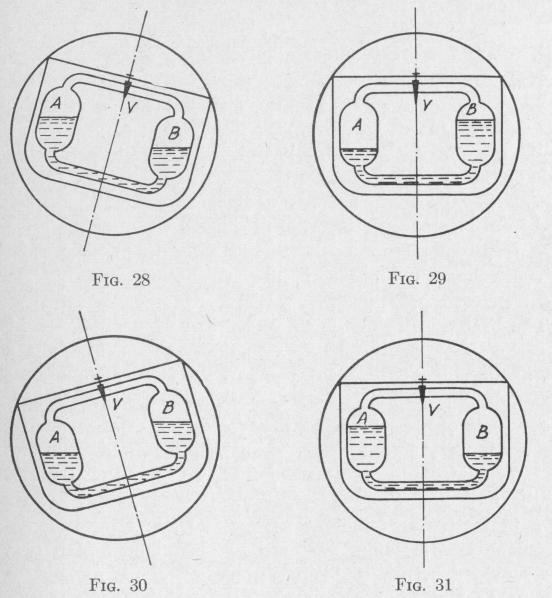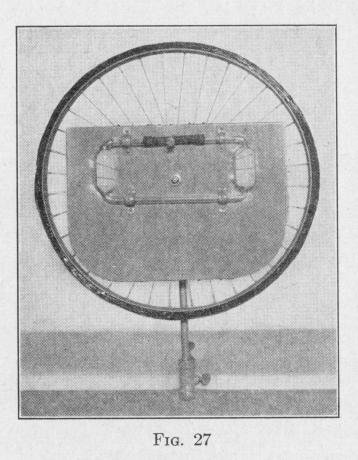Previous Page Index(For best results view this page as a PDF) Next Page

40   PRINCIPLES OF ELEMENTARY DYNAMICS

If the pendulous system be tilted, and held in this tilted position,

liquid will flow from the higher tank to the lower, and thereafter

will flow back and forth several times with a definite period. If

the pendulous system be set into oscillation, and the frequency of

this oscillation be nearly the same as that of the liquid, then by the

time that the system is in its equilibrium position, there will be

an excess of liquid in the reservoir which was the lower when the

system was displaced from the equilibrium position. Thus, there will be a difference in phase between the oscillation of the pendulous system and the oscillation of the liquid in the tanks.

The period of oscillation of the liquid, as well as the phase difference between the oscillations of the liquid and of the pendulous system, can be regulated by adjusting a valve V in either of the tubes that connect the two tanks. If the period of flow of liquid back and forth equals the period of the pendulous system, and

if the velocity of the liquid is a quarter period ahead of that of

the pendulous system, then the following effects will occur.

Consider the action at an instant when the pendulous system has

a large deflection, and there are equal amounts of liquid in the two

tanks, Fig. 28. While the pendulous system is moving from this

position to the equilibrium position, an excess of liquid rises in the

tank that was the lower, the excess being maximum when the

pendulous system is in the equilibrium position, Fig. 29. During

this quarter period, the distance between the center of mass of the

pendulous system and the vertical line through the axis of vibra

tion is being diminished. Hence, the torque urging the pendulous

system toward the equilibrium position is being diminished faster

than it would be if the liquid were not flowing.

While the pendulous system is moving from the equilibrium

position, Fig. 29, to the end of the swing, Fig. 30, the excess of

SIMPLE HARMONIC MOTION

liquid in the tank on the advancing side of the pendulous system produces a torque that opposes the motion of the pendulous system.

By the time that the pendulous system again has attained its equilibrium position, a maximum excess of liquid has flowed into the tank A, Fig. 31, which was the lower in Fig. 30. During motion, the torque urging the pendulous system towards the equilibrium position is being diminished faster than it would be if the

liquid were not flowing. Consequently, the amplitude of swing is less than it would be if the liquid were not flowing.

Thus, the amplitude of vibration of the pendulous system is being damped throughout the half cycle while the system is moving from its equilibrium position to the end of its path and back. During the next half cycle the amplitude of vibration is damped in the same way. The damping of the amplitude of vibration involves abstraction of energy from the vibrating pendulous system. This energy is transformed into heat as the liauid and airPrevious Page Index(For best results view this page as a PDF) Next Page